You are currently offline. Some features of the site may not work correctly.

# Polyhedral graph

In geometric graph theory, a branch of mathematics, a polyhedral graph is the undirected graph formed from the vertices and edges of a convex… Expand
Wikipedia

## Papers overview

Semantic Scholar uses AI to extract papers important to this topic.
2013
2013
• IMR
• 2013
• Corpus ID: 721691
We present a preliminary method to generate polyhedral meshes of general non-manifold domains. The method is based on computing… Expand
•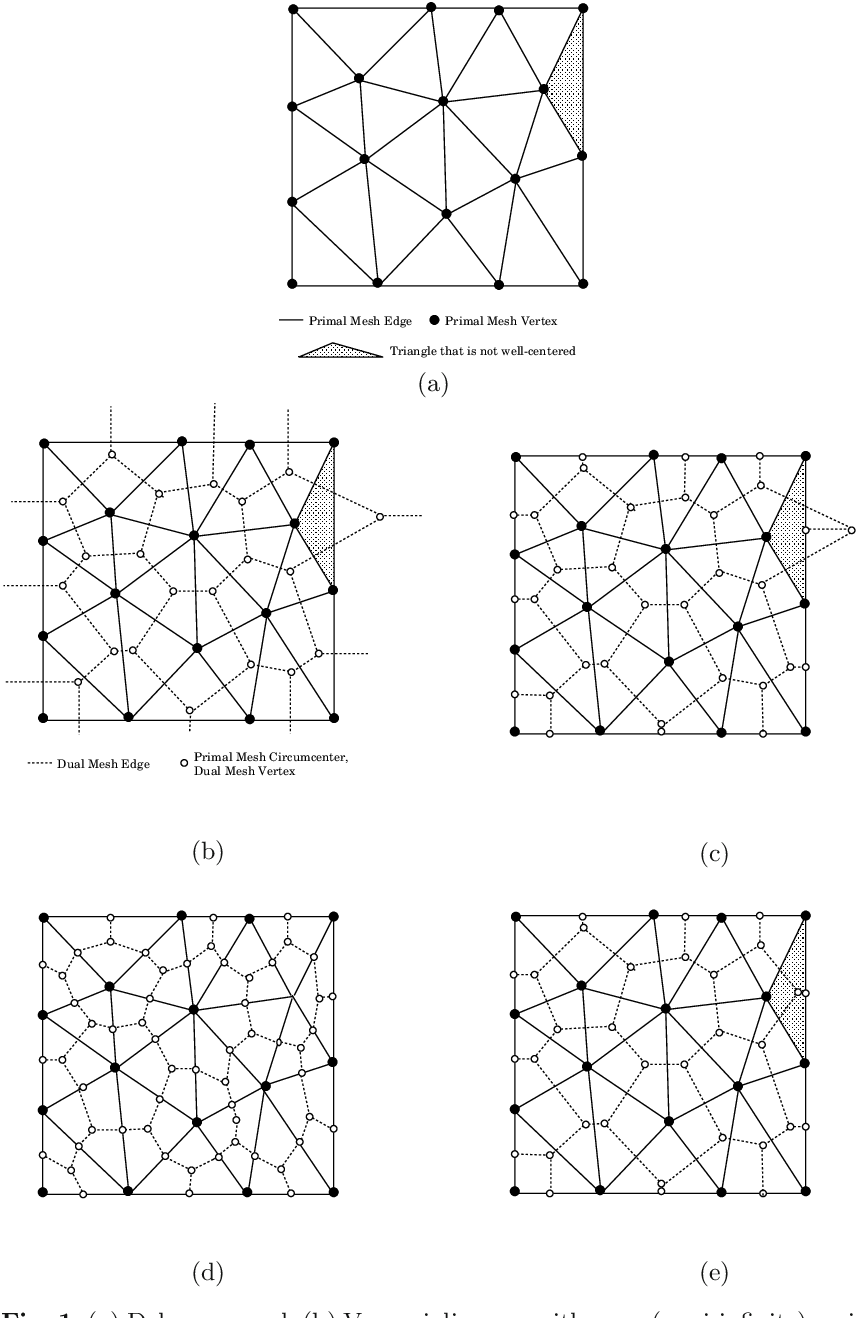•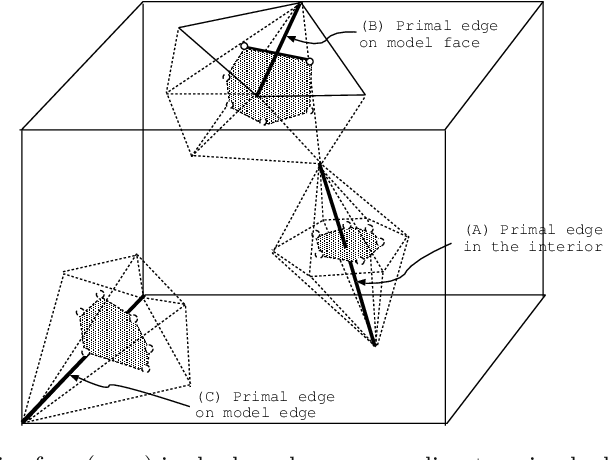•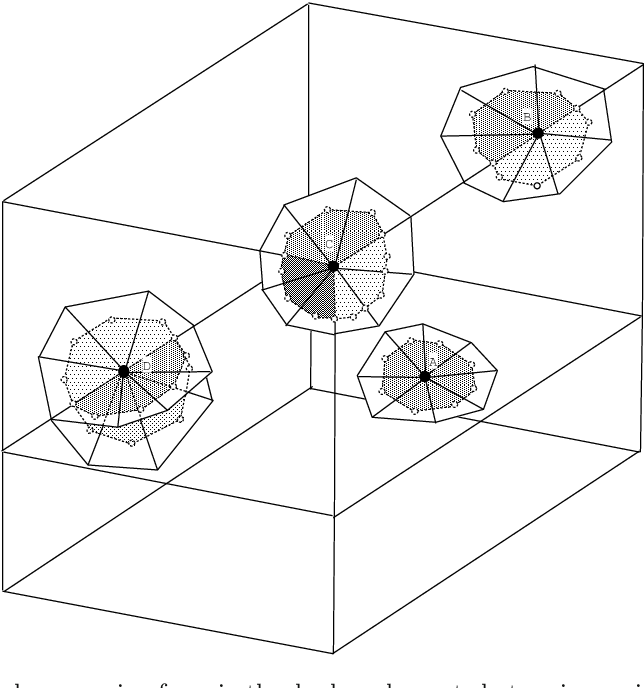•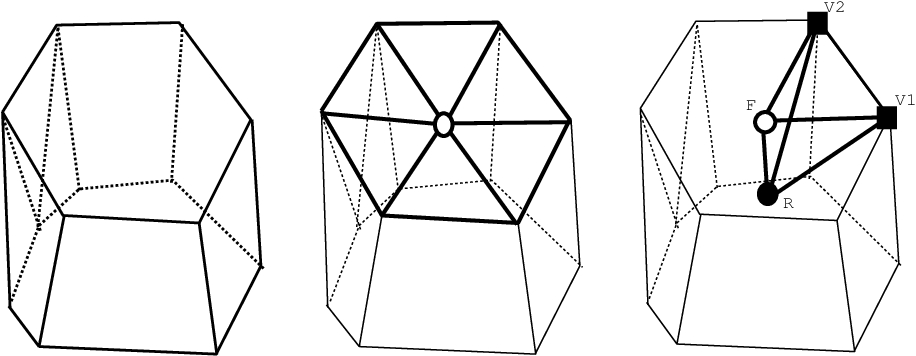•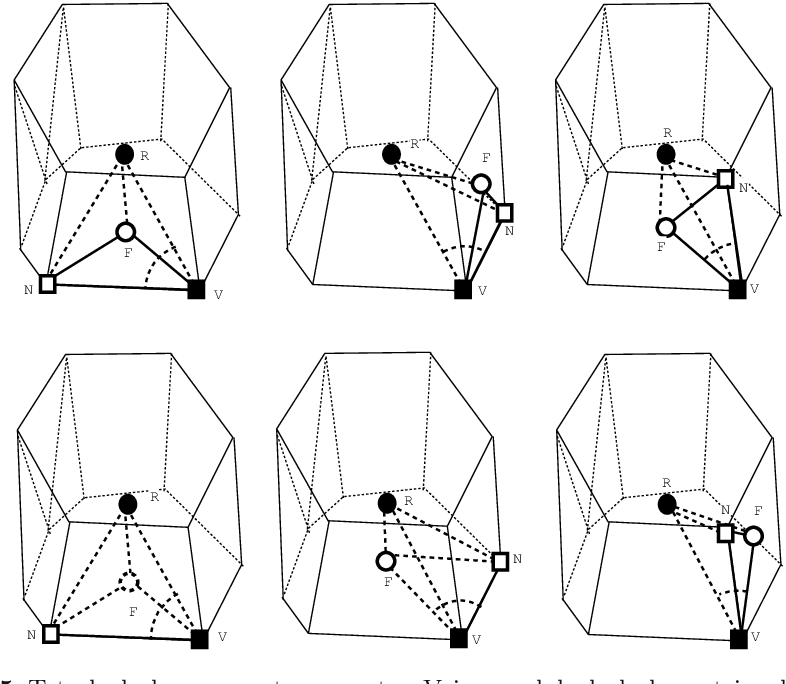Is this relevant?
2011
2011
• Edward D. Kim
• Electron. Notes Discret. Math.
• 2011
• Corpus ID: 14918924
Abstract We introduce a new combinatorial abstraction for the graphs of polyhedra. The new abstraction is a flexible framework… Expand
•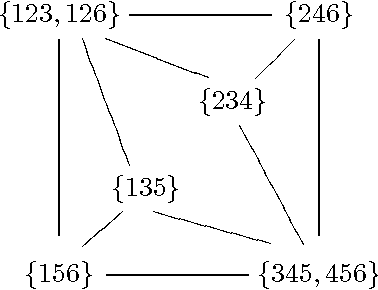•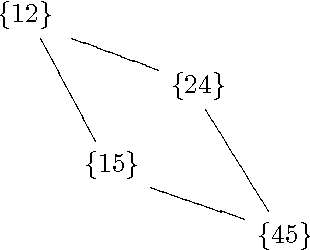•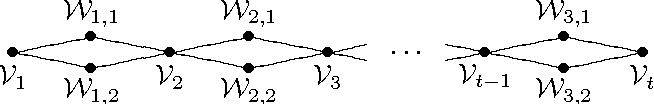•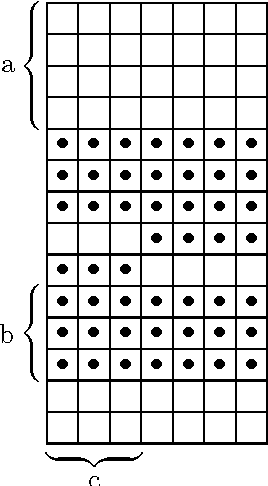Is this relevant?
2010
2010
• Discret. Math.
• 2010
• Corpus ID: 81420
A graph H is defined to be light in a family H of graphs if there exists a finite number @f(H,H) such that each G@?H which… Expand
•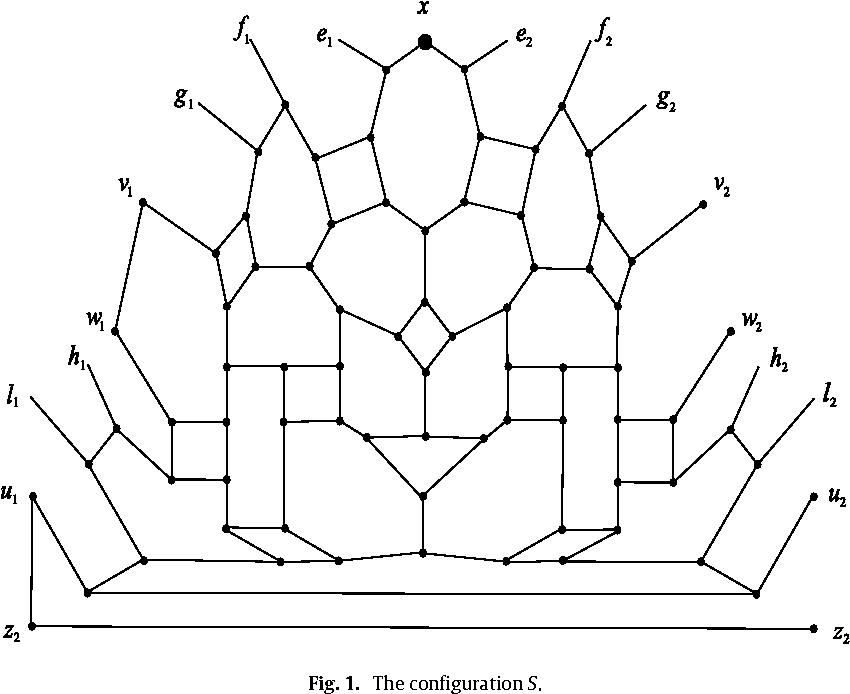•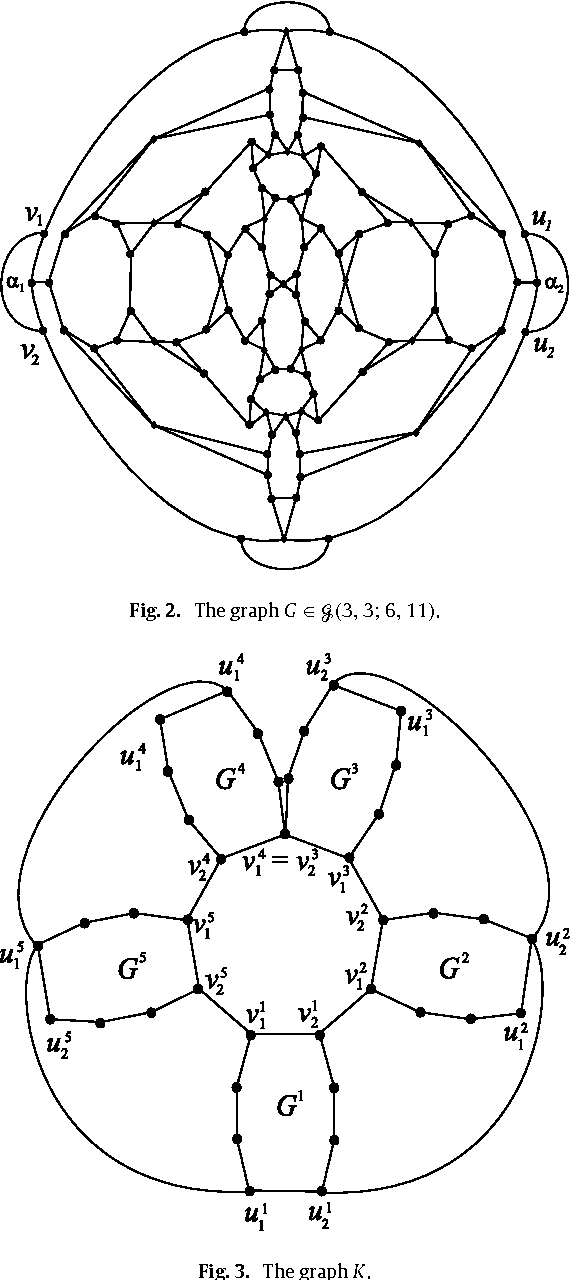•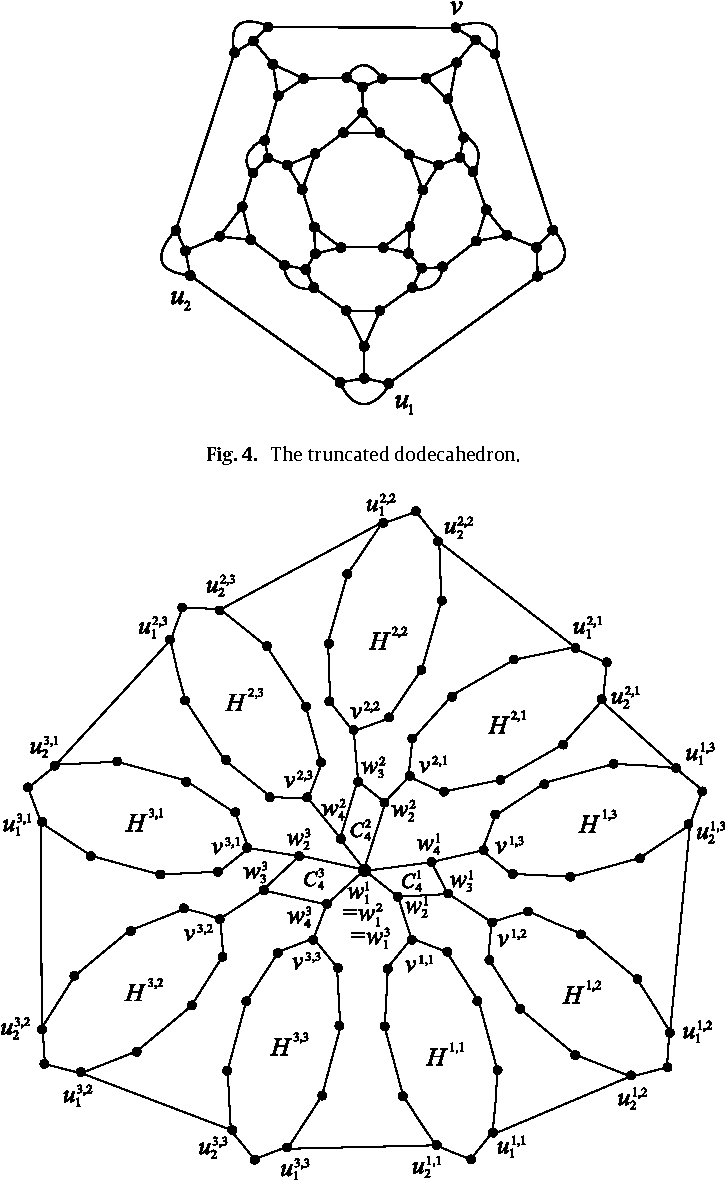•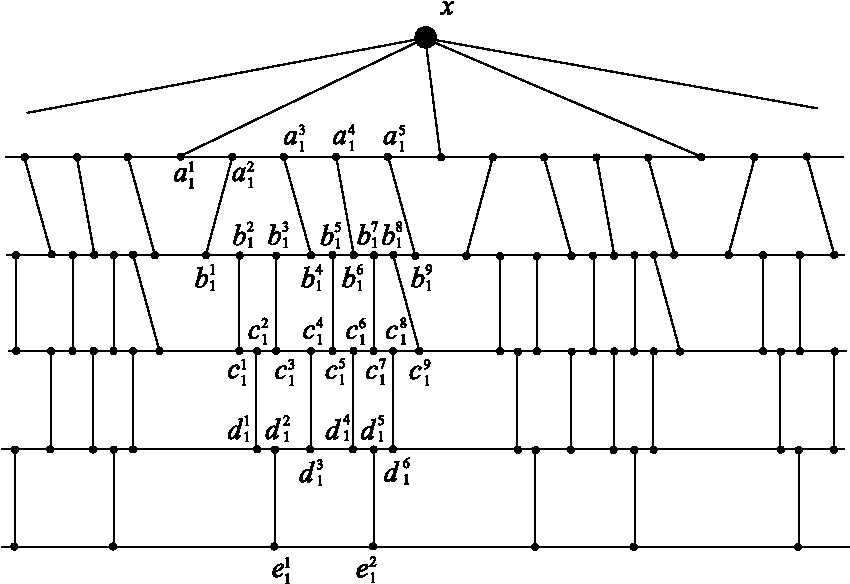•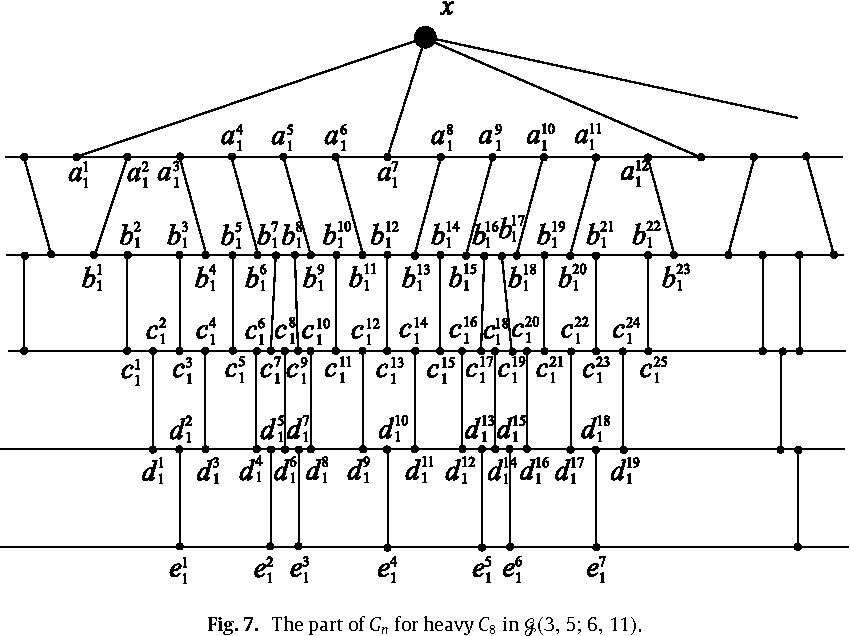Is this relevant?
2007
2007
• Journal of Graph Theory
• 2007
• Corpus ID: 5296920
Let G be a 3-connected planar graph and G∗ be its dual. We show that the pathwidth of G∗ is at most 6 times the pathwidth of G… Expand
•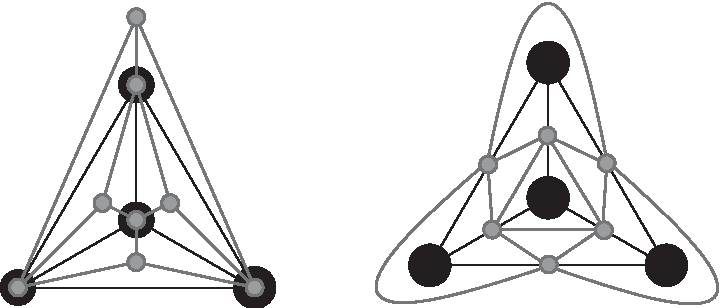•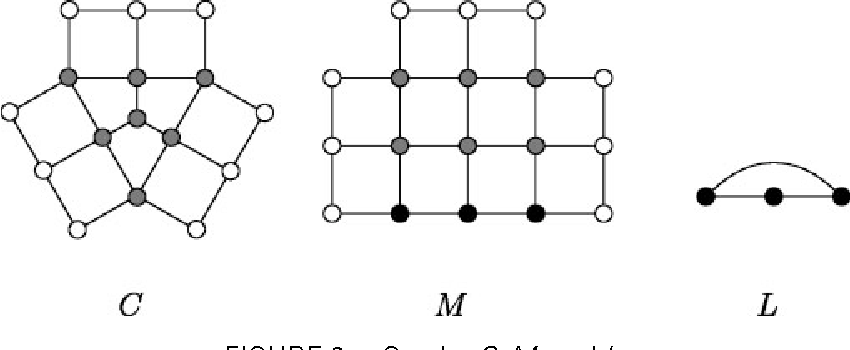•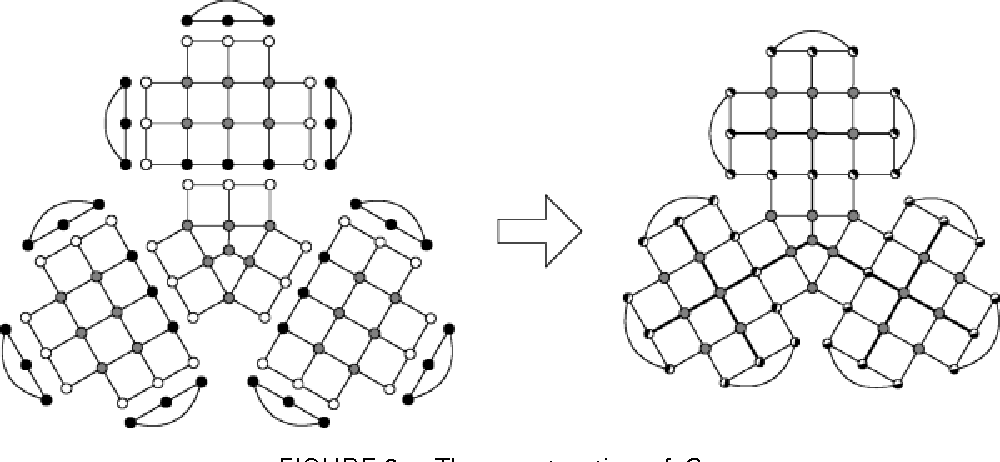Is this relevant?
2005
2005
• 2005
• Corpus ID: 124055621
•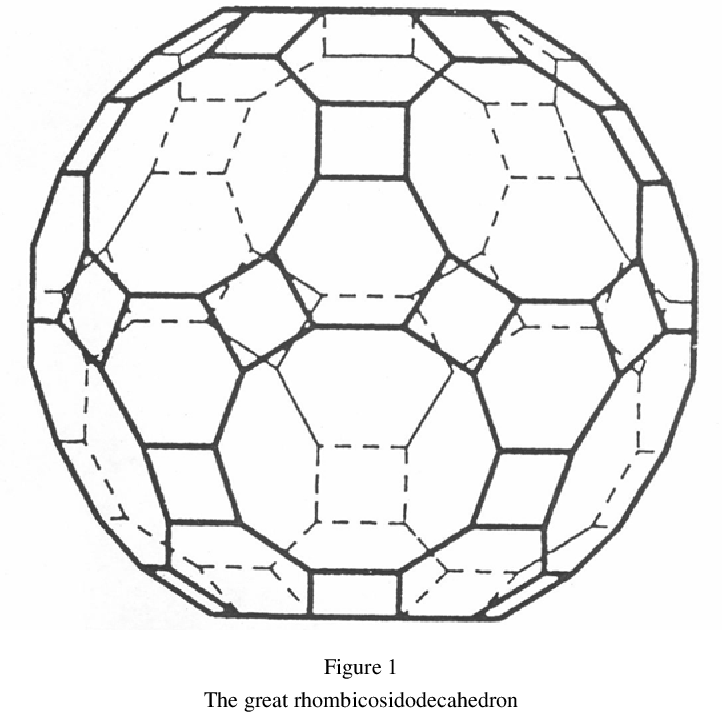•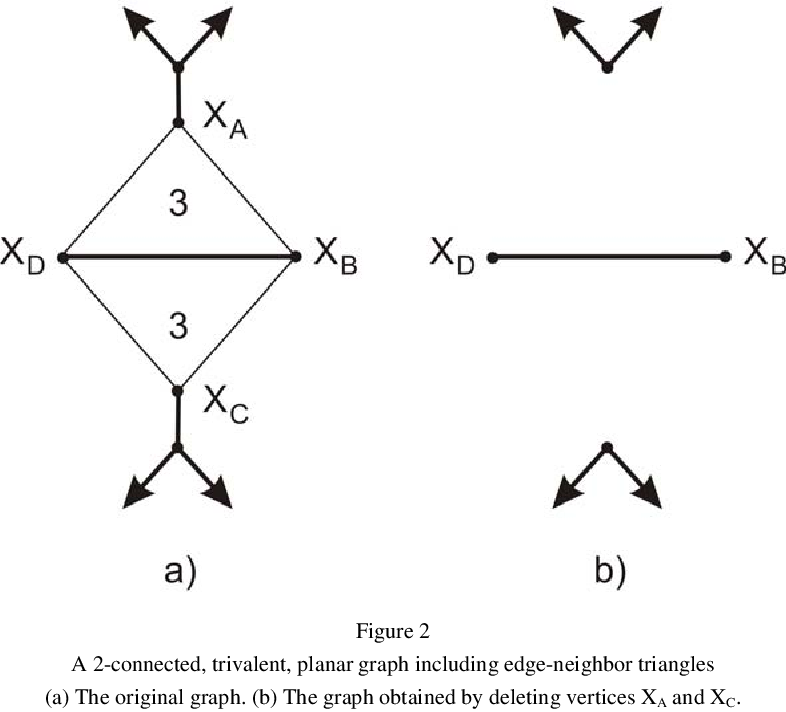•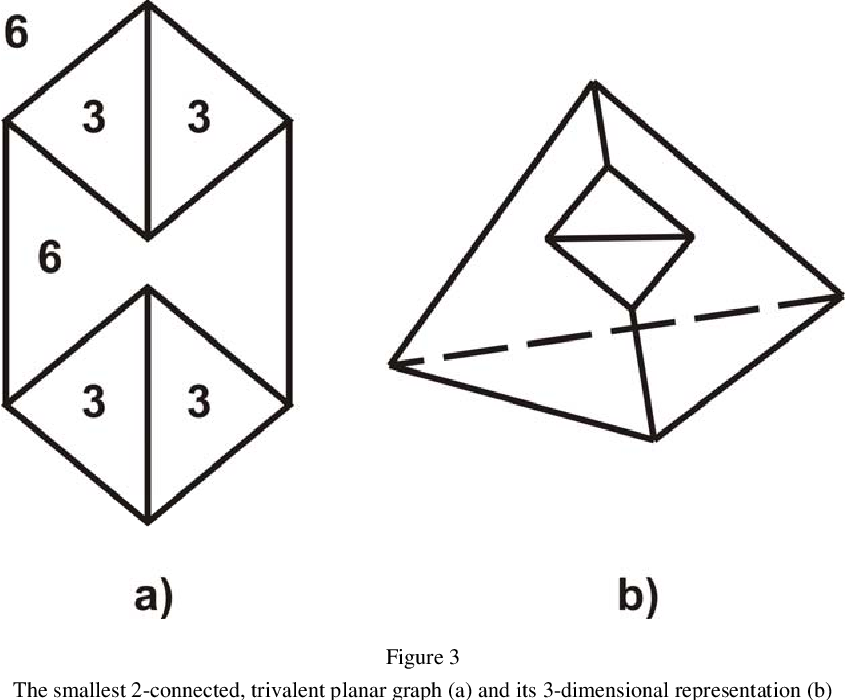•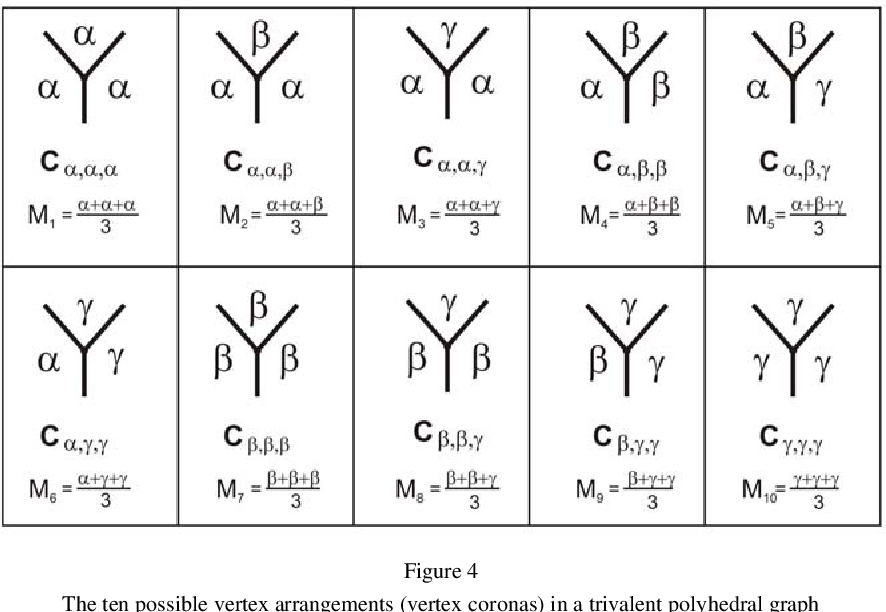•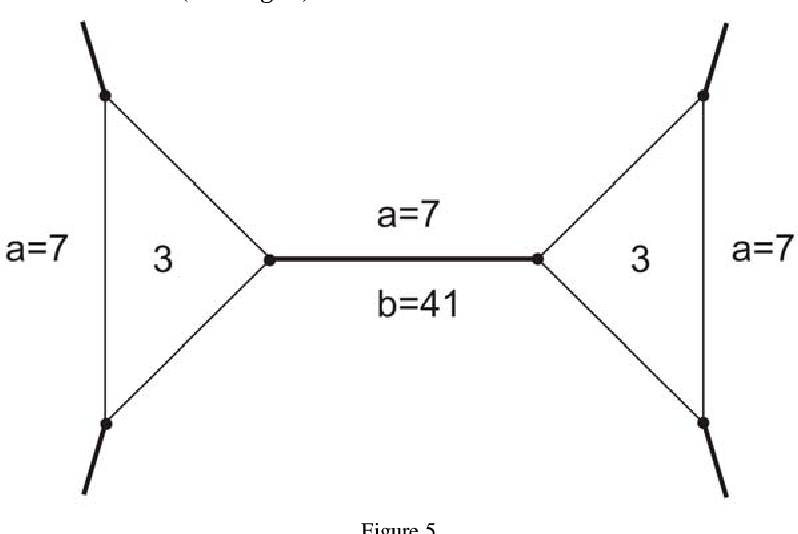Is this relevant?
2004
2004
• Discret. Appl. Math.
• 2004
• Corpus ID: 31608409
Let G = G(V,E,F) be a polyhedral graph with vertex set V, edge set E and face set F. e = (x, y; α, β) ∈ E(G) denotes an edge… Expand
Is this relevant?
2000
2000
• Discret. Math.
• 2000
• Corpus ID: 9375818
Abstract It is proved that every 3-connected planar graph G with δ ( G )⩾4 either does not contain any path on k ⩾8 vertices or… Expand
•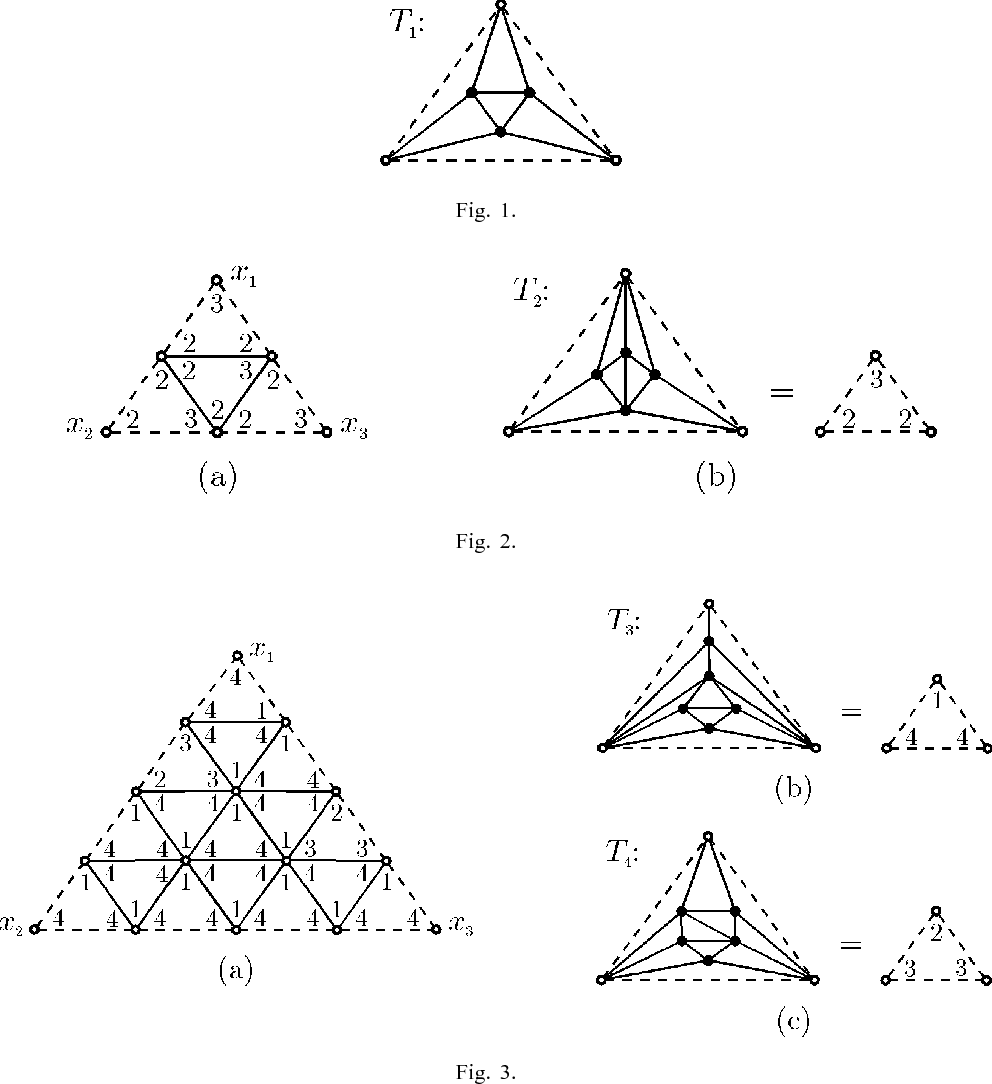•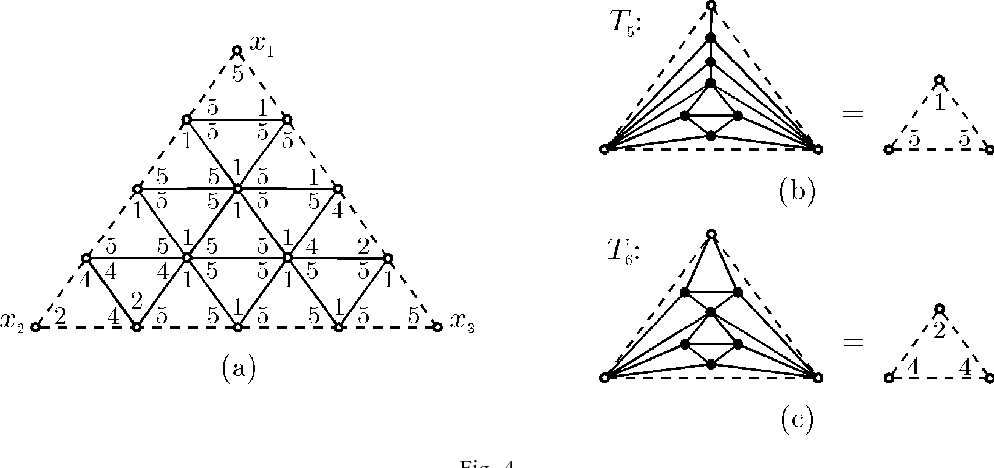•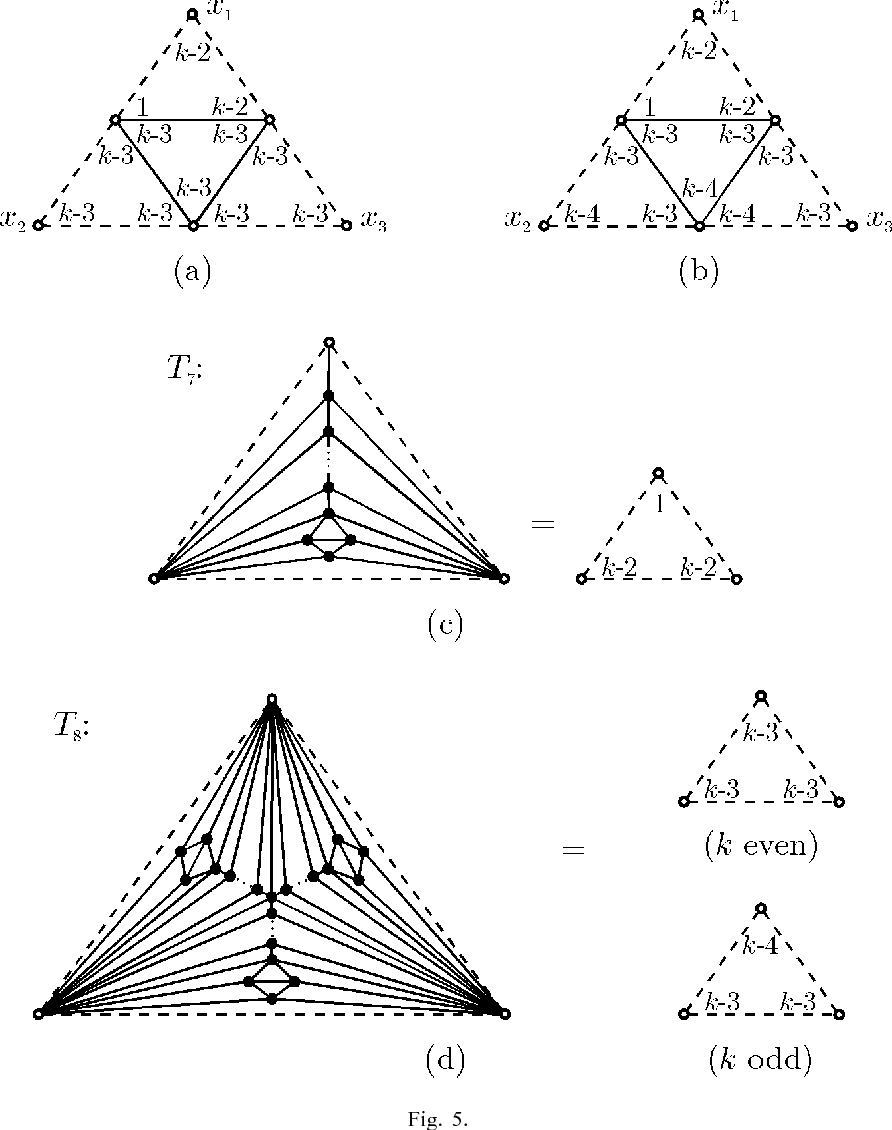•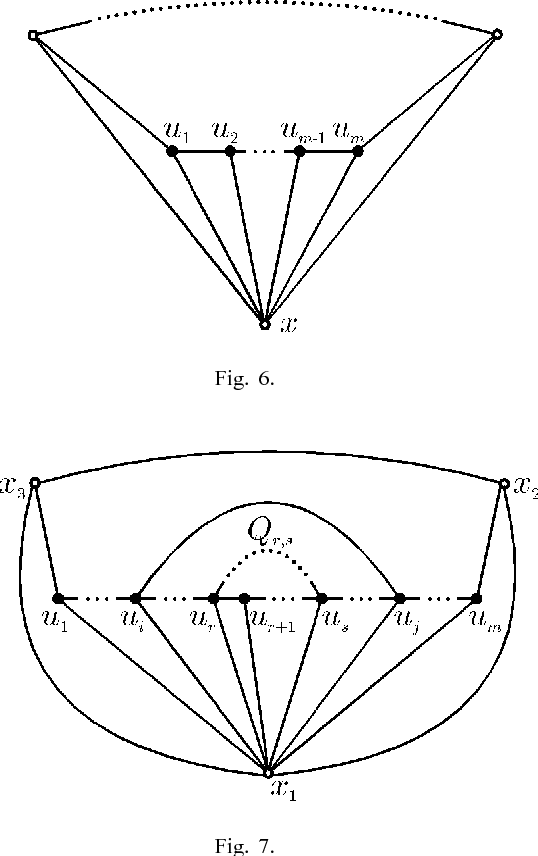•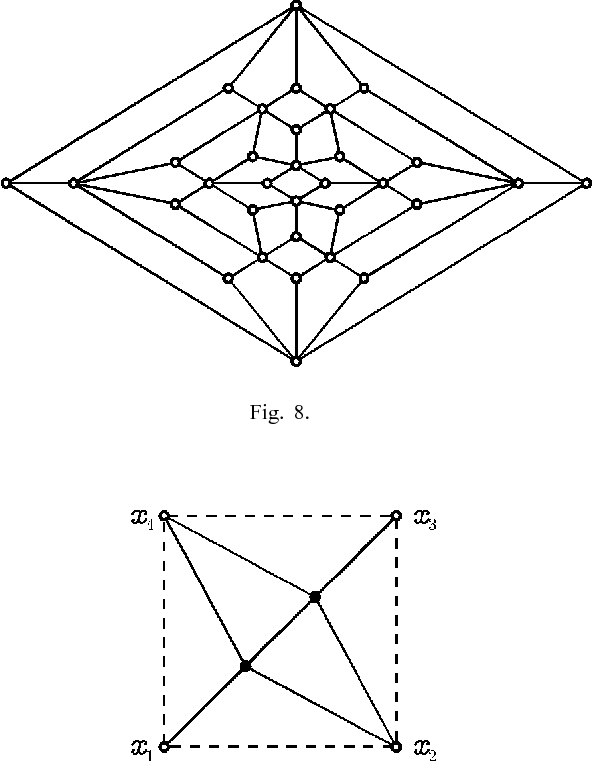Is this relevant?
1999
1999
It is proved that each polyhedral graph that contains a vertex of degree at least 52k, contains also a path Pk on k vertices such… Expand
Is this relevant?
1993
1993
Abstract By a theorem of W.T. Tutte the toughness t ( G ) of a nonhamiltonian polyhedral graph G is less than or equal to 3/2… Expand
Is this relevant?
1973
1973
• Discret. Math.
• 1973
• Corpus ID: 263276
It is shown that every group of sense preserving automorphisms of a polyhedral graph is isomorphic to a group of rotations of the… Expand
Is this relevant?